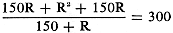Please Support RF Cafe by purchasing my  ridiculously low−priced products, all of which I created.

RF Workbench  (shareware)August 1963 Radio-Electronics[Table of Contents] Wax nostalgic about and learn from the history of early electronics. See articles from Radio-Electronics, published 1930-1988. All copyrights hereby acknowledged.

For a couple years in the 1963 era, Radio−Electronics magazine ran a monthly feature called "What's Your EQ?," where EQ stood for Electronics Quotient. It was a play on IQ - Intelligence Quotient. Some the problems posed are real posers, but most are within the capability of RF Cafe visitors - even the ones showing vacuum tubes since you can usually mentally replace them with transistors and solid state diodes. "L-Pad Puzzler" is a fairly simple circuit analysis equation. Be sure to approach it from terminals 3 and 4. The solution given for "Fussy Fuses" is questionable the way I look at it. It seems to me if the five branches have no resistance (or even exactly the same resistance), the current would split equally amongst them, so as current is increased the 1A would blow, then the 2A, up through the 5A, then that would be it. R−E concluded differently. "Voltage Divider" is is a little more of a challenge.Three puzzles for the student, theoretician and practical man. They may look simple, but double-check your answers before you say you've solved them. If you've got an interesting or unusual answer send it to us. We are especially interested in service stinkers or engineering stumpers on actual electronic equipment. We are getting so many letters we can't answer individual ones, but we'll print the more interesting solutions (the ones the, original authors never thought of). We will pay \$10 and up for each one accepted. Write EQ Editor, Radio-Electronics, 154 West 14th Sr., New York, N.Y.

Two equal resistors form an L-pad. When a load of 150 ohms is connected to terminals 1 and 2, an ohmmeter shows 300 ohms between terminals 3 and 4. What are the values of the resistors?

- Kendall Collins

The number next to each fuse is its current rating in amperes. What current will this fuse combination carry?

- Steve Stumph

After charging current stops, what are the voltages across C1 , C2 and C3?

- Cleve G. Cleveland

Answers for this month's puzzlers are on page 67.

Quizzes from vintage electronics magazines such as Popular Electronics, Electronics-World, QST, and Radio News were published over the years - some really simple and others not so simple. Robert P. Balin created most of the quizzes for Popular Electronics. This is a listing of all I have posted thus far.

With no load across terminals 1 and 2, an ohmmeter across 3 and 4 will read just 2R - the two equal resistors in series. When we connect 150 ohms between terminals 1 and 2, we shunt one of the two equal resistors, and the total resistance "seen" now from 3 and 4 isJust following the rules of algebra, we can solve the R very easily by putting both terms on the left of the equals sign over a common denominator, 150 +R:When we simplify and remove the parentheses, we getNow multiply both sides by 150 + R, to get rid of the denominator:

R2 + 300R = 45,000 + 300R

We can throw out the term 300R, since it appears with the same sign on both sides of the equals sign. That leaves just R2 = 45,000, or R = 212.5 ohms.

Fussy Fuses

The 6-amp through 10-amp fuses are large enough that their current-carrying capacity does not determine the maximum current of the circuit and are used only to make the reader think. Each fuse in parallel will carry the same amount of current, since each fuse offers no practical resistance to current flow. Therefore, if the circuit carries more than 5 amps of current, the 1-amp fuse will blow. More than 8 amps will blow the 2-amp fuse, and more than 9 amps will blow the 3-amp fuse, after which the 4-amp and the 5-amp fuses will blow.

So the combination carries 9 amps.

Voltage Divider?

After charging current stops, the voltage across the resistors must be in proportion to the resistors. Thus there will be 270 volts across the 90,000-ohm resistor, and 30 volts across the 10,000-ohm resistor. Therefore, if the voltage across C2 equals x, that across C1 must equal 300 - x, and across C3, x - 30.

Since charging current for C2 and C3 is drawn from C1, Q1 = Q2 + Q3. Q is charge, and Q = CV.

Therefore: 1 * (300 - x) = 2 (x) + 3 * (x - 30)

Remove parentheses: 300 - x = 2x + 3x - 90, 6x = 390, and x = 65.

Therefore, voltage on C1 = 235, voltage on C2 = 65, and voltage on C3 = 35.

Posted June 23, 2023